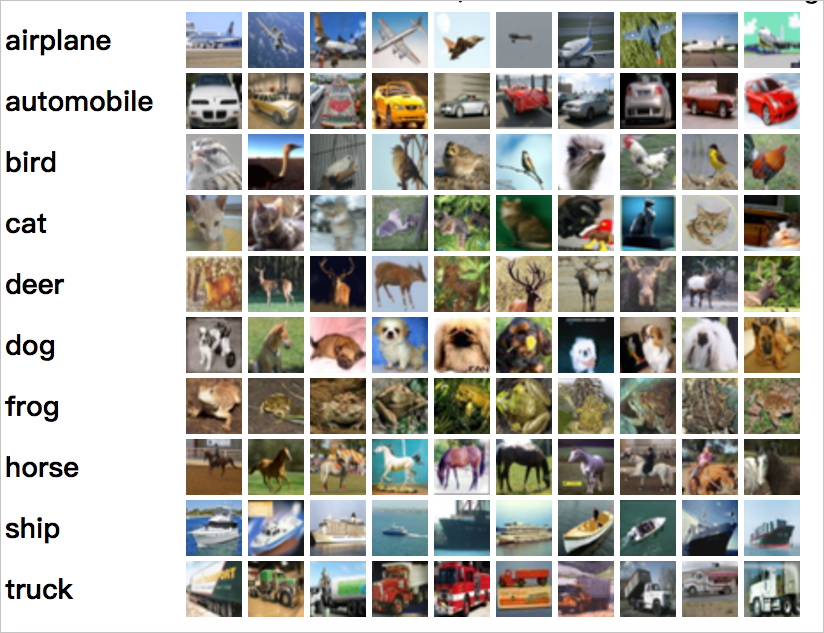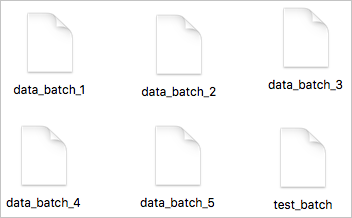This topic describes how to use Machine Learning Platform for AI (PAI)-TensorFlow to build an image classification model.

## Prerequisites

• An Object Storage Service (OSS) bucket is created. PAI is authorized to access the OSS bucket. For more information, see Create buckets and OSS authorization.
Notice When you create an OSS bucket, make sure that versioning is disabled for the bucket. Otherwise, model training may fail.
• Graphics processing unit (GPU) is enabled for the PAI project where you want to build the model. For more information, see GPU resource authorization.

## Background information

As the Internet develops, masses of images and voice data have been generated. Such unstructured data is difficult to be mined and effectively used due to the following reasons:
• Deep learning algorithms are required, which are difficult to learn.
• GPU compute engines are required, which causes high cost.

Machine Learning Studio presets a template that uses a deep learning framework to build image classification models. You can create an experiment by using the template and reuse the experiment for other purposes such as pornographic image detection, facial recognition, and object detection.

## Dataset

In the following sample experiment, the CIFAR-10 dataset is used. The CIFAR-10 dataset contains 60,000 color images of 32 × 32 pixels. The images fall into 10 categories, including airplane, automobile, bird, cat, deer, dog, frog, horse, ship, and truck, as shown in the following figure. For more information about the download URLs of the dataset and relevant code, see CIFAR-10 dataset.In the experiment, the dataset is divided into a training dataset of 50,000 images and a prediction dataset of 10,000 images. The training dataset is further divided into five training batches. The entire prediction dataset is used as a prediction batch. Each batch is a separate file, as shown in the following figure.## Prepare data

Upload the dataset files and relevant code that are used for this experiment to an OSS bucket. For example, you can create the aohai_test folder in an OSS bucket and four subfolders in the aohai_test folder, as shown in the following figure.The four subfolders are used for different purposes:
• check_point: stores the model that is generated by the experiment.
Note After you create an experiment by using the preset template in Machine Learning Studio, you must set the Checkpoint Output Directory/Model Input Directory parameters of the TensorFlow components to the path of an existing OSS folder. Otherwise, you cannot run the experiment. In this experiment, set the Checkpoint Output Directory/Model Input Directory parameters of the TensorFlow components to the path of the check_point subfolder.
• cifar-10-batches-py: stores the cifar-10-batcher-py file of the training dataset and the bird_mount_bluebird.jpg file of the prediction dataset.
• train_code: stores the cifar_pai.py file, which contains the training code.
• predict_code: stores the cifar_predict_pai.py file, which contains the prediction code.

## Procedure

1. Go to a Machine Learning Studio project.
1. Log on to the Machine Learning Platform for AI (PAI) console.
2. In the left-side navigation pane, choose Model Training > Visualized Modeling (Machine Learning Studio).
3. In the upper-left corner of the page, select the region that you want to manage.
4. Optional:In the search box on the PAI Visualization Modeling page, enter the name of a project to search for the project.
5. Find the project and click Machine Learning in the Actions column.
2. Create an experiment.
1. In the left-side navigation pane, click Home.
2. In the Templates section, click Create below Tensorflow image classification.
3. In the New Experiment dialog box, set the parameters as required. You can use the default values for the parameters.
Parameter Description
Name The name of the experiment. Default value: Tensorflow image classification.
Project The name of the project to which the experiment belongs. You cannot change the value of this parameter.
Description The name of the experiment. Default value: Use PAI-Tensorflow to build an image classification model.
Save To The directory for storing the experiment. Default value: My Experiments.
4. Click OK.
5. Optional:Wait about 10 seconds. In the left-side navigation pane, click Experiments.
6. Optional:Click TensorFlow image classification_XX under My Experiments.
My Experiments is the directory for storing the experiment that you created and TensorFlow image classification_XX is the name of the experiment that you created. In the experiment name, _XX is the ID that the system automatically creates for the experiment.
7. View the components of the experiment on the canvas, as shown in the following figure. The system automatically creates the experiment based on the preset template.Area No. Description
1 The component in this area reads the training data. The system automatically sets the OSS Data Path parameter of this component to the path of the training dataset that this experiment uses. If you want to use another dataset for the training, click the Read File Data-1 component on the canvas. In the Fields Setting pane on the right, set the OSS Data Path parameter to the OSS path of the training dataset that you want to use.
2 The component in this area reads the prediction data. The system automatically sets the OSS Data Path parameter of this component to the path of the prediction dataset that this experiment uses. If you want to use another dataset as the prediction dataset, click Read File Data-2 on the canvas. In the Fields Setting pane on the right, set the OSS Data Path parameter to the OSS path of the prediction dataset that you want to use.
3 The component in this area trains the model by using PAI-TensorFlow. For this component, set the Checkpoint Output Directory/Model Input Directory parameter and use the default values for other parameters. The following parameters specify the OSS paths of the corresponding files or folders:
• Python Code Files: the OSS path of the cifar_pai.py file.
• Data Source Directory: the OSS path of the cifar-10-batches-py folder. The system automatically synchronizes data from the upstream Read File Data-1 component.
• Checkpoint Output Directory/Model Input Directory: the OSS path of the check_point folder, which stores the model that is generated.
4 The component in this area generates the prediction result. For this component, set the Checkpoint Output Directory/Model Input Directory parameter and use the default values for other parameters. The following parameters specify the OSS paths of the corresponding files or folders:
• Python Code Files: the OSS path of the cifar_predict_pai.py file.
• Data Source Directory: the OSS path of the cifar-10-batches-py folder. The system automatically synchronizes data from the upstream Read File Data-2 component.
• Checkpoint Output Directory/Model Input Directory: the OSS path of the check_point folder, which stores the model that is generated. This parameter must be set to the same value as the Checkpoint Output Directory/Model Input Directory parameter of the component that trains the model by using PAI-TensorFlow.
3. Run the experiment and view the result.
1. In the top toolbar of the canvas, click Run.
2. After the experiment is run, you can view the prediction result that is stored in the specified OSS path(Checkpoint Output Directory/Model Input Directory).

## Training code

This section describes the key code in the cifar_pai.py file.
• The following code can be used to train a convolutional neural network (CNN) model for image classification:
``````network = input_data(shape=[None, 32, 32, 3],
data_preprocessing=img_prep,
data_augmentation=img_aug)
network = conv_2d(network, 32, 3, activation='relu')
network = max_pool_2d(network, 2)
network = conv_2d(network, 64, 3, activation='relu')
network = conv_2d(network, 64, 3, activation='relu')
network = max_pool_2d(network, 2)
network = fully_connected(network, 512, activation='relu')
network = dropout(network, 0.5)
network = fully_connected(network, 10, activation='softmax')
loss='categorical_crossentropy',
learning_rate=0.001)``````
• The following code can be used to generate a model named model.tfl:
``````    model = tflearn.DNN(network, tensorboard_verbose=0)
model.fit(X, Y, n_epoch=100, shuffle=True, validation_set=(X_test, Y_test),
show_metric=True, batch_size=96, run_id='cifar10_cnn')
model_path = os.path.join(FLAGS.checkpointDir, "model.tfl")
print(model_path)
model.save(model_path)``````

## Prediction code

This section describes the key code in the cifar_predict_pai.py file. First, the system reads the bird_bullocks_oriole.jpg image file and resizes the image to 32 × 32 pixels. Then, the system passes the image to the model.predict function. The output of the function is the weights of the 10 categories as which the image is recognized: ['airplane','automobile','bird','cat','deer','dog','frog','horse','ship','truck']. Finally, the system returns the category with the highest weight as the prediction result.
``````    predict_pic = os.path.join(FLAGS.buckets, "bird_bullocks_oriole.jpg")